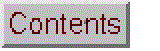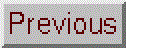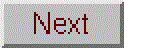# Instrumental Deviation from Beer's Law

### Excel and OpenOffice Calc Versions

 Screen animation of version 1. Click to enlarge.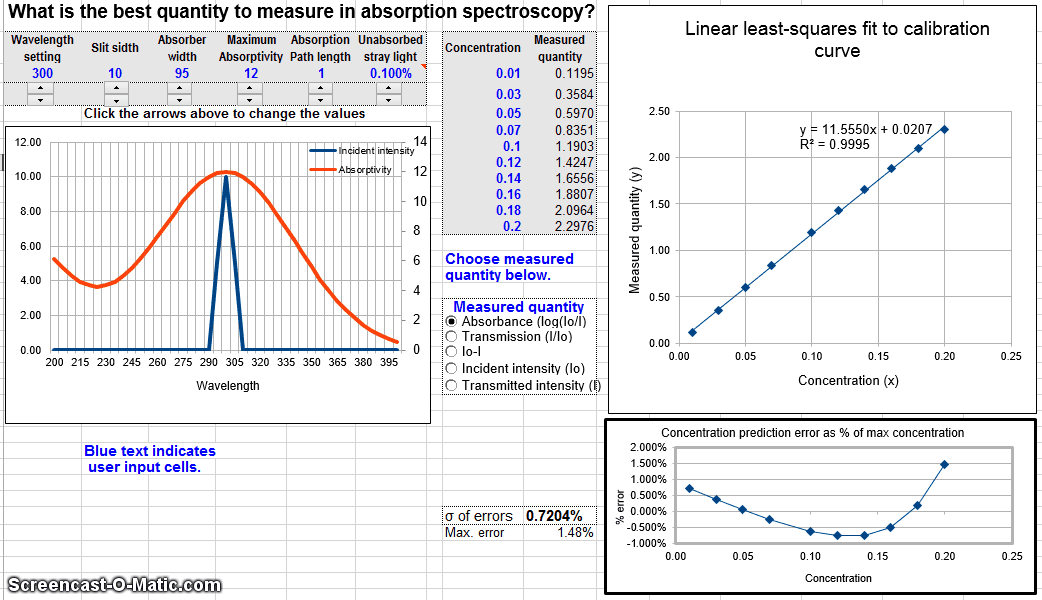Screen animation of version 2. Click to enlarge.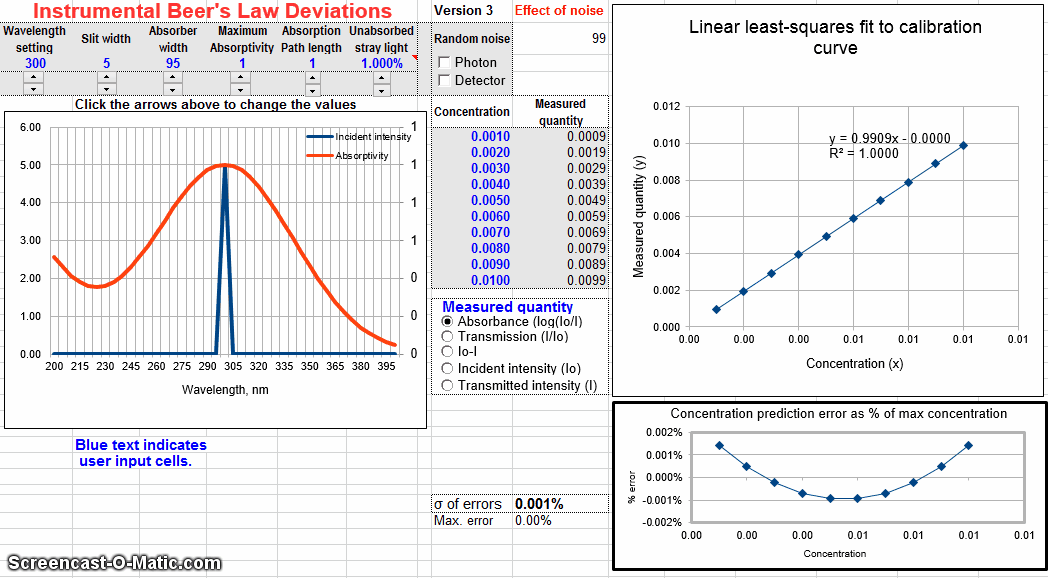Screen animation of version 3. Click to enlarge.

[Assumptions]   [Background]   [Frequently Asked Questions]   [Student handout]   [WingZ version]

This spreadsheet is a numerical simulation of absorption spectroscopy. It computes the measured absorbance and plots the analytical curve (absorbance vs concentration) for a simulated absorber measured in an absorption spectrophotometer with variable wavelength, spectral bandpass and unabsorbed stray light, given the maximum absorptivity, path length, and half-width of the absorber, and the slit width and percent unabsorbed stray light of the monochromator. The arrow buttons below each of these parameters allow you to change the values quickly without typing. The spectra and the analytical curve change dynamically as the variables are changed. Any list of concentrations can be typed in for the analytical curve. The spreadsheet fits a straight line to the calibration curve and calculates the slope, intercept, the correlation coefficient R2, and the percent relative error in predicting concentrations from the fitted line.

Alternative versions: Version 1 is the basic (simplest) version. The optional Version 2 allows the user to select which quantity to plot vs concentration: absorbance (log(Io/I)), transmission (I/Io), absorbed intensity (Io-I), or I and Io separately. This version can be used to demonstrate the utility of computing absorbance. Version 3 includes optional random noise in the measurement of light intensity (photon and/or detector noise), which is more realistic.

Assumptions of this simulation: The true monochromatic absorbance is assumed to follow the Beer-Lambert Law; the absorber spectrum consists of two peaks, at fixed wavelengths of 150 and 300 nm, that have either Gaussian or Lorentzian shape (selectable by user); the spectral width of the light source is much greater than the monochromator spectral bandpass; the monochromator has a triangular slit function (i.e. the entrance and exit slits are equal in width and are rectangular in shape); the absorption path length and absorber concentration are both uniform across the light beam; the spectral response of the detector is much wider that the spectral bandpass of the monochromator. Only version 3 includes the effect of random noise (see Signal-to-noise ratio of absorption spectrophotometry, Effect of Slit Width on Signal-to-Noise Ratio in Absorption Spectroscopy, and Comparison of Calibration Curve Fitting Methods in Absorption Spectroscopy for other simulations that include random noise).

Note: In the quantitative analysis of known absorbers, these instrumental deviations from Beer's Law can be avoided computationally by applying curve-fitting to the spectra, rather than to the calibration curve, using the Transmission Fitting (TFit) Method.

View Equations (.pdf)
; in Excel format (.xls)
; in Excel format (.xls)

WingZ Versions

Other related simulations:
Comparison of Calibration Curve Fitting Methods in Absorption Spectroscopy
Signal-to-noise ratio of absorption spectrophotometry
Effect of Slit Width on Signal-to-Noise Ratio in Absorption Spectroscopy
Error propagation in Analytical Calibration
Spectroscopic Simulation of Atomic Absorption

Worksheets for linear and non-linear calibration curves for your own data
The Transmission Fitting (TFit) Method applies curve fitting to the spectra rather than the calibration data.

Background

The Beer-Lambert Law. In absorption spectroscopy, the intensity I of light passing through an absorbing sample is given by the Beer-Lambert Law:

I = Io*10-(alpha*L*c)

where “Io” is the intensity of the light incident on the sample, “alpha” is the absorption coefficient or the absorptivity of the absorber, “L” is the distance that the light travels through the material (the path length), and “c” is the concentration of absorber in the sample. The variables I, Io, and alpha are all functions of wavelength; L and c are scalar. In conventional applications, measured values of I and Io are used to compute the absorbance, defined as

A = log(Io/I) = alpha*L*c

Absorbance defined in this way is (ideally) proportional to concentration, which simplifies analytical calibration.

The absorption coefficient alpha is determined experimentally.  If you solve the above equation for alpha, you get:

alpha = A/(L*c)

So by measuring the absorbance A of a known concentration c of the absorbing compound using an absorption path length L, you can calculate alpha. Because A has no units (it's the log of a ratio of two intensities, so the intensity units cancel out), the units of  alpha are the reciprocal of the units of  L and c.  For example, if the path length L is in cm and the concentration c is in moles/liter, alpha is in liters/mole-cm.  Even better is to prepare a series of solutions at different concentrations and plot the measured absorbances vs the concentrations; the resulting plot is called a calibration curve or analytical curve.  The slope of this curve is alpha*L, so if you measure the slope and divide by L, you have alpha.

Deviations from the Beer-Lambert Law. It's important to understand that the "deviations" from the Beer-Lambert Law discussed here are not actually failures of this law but rather apparent deviations caused by failures of the measuring instrument to adhere to the condition under which the law is derived. The fundamental requirement under which then Beer-Lambert Law is derived is that every photon of light striking the detector must have an equal chance of absorption. Thus, every photon must have the same absorption coefficient alpha, must pass through the same absorption path length, L, and must experience the same absorber concentration, c. Anything that upsets these conditions will lead to an apparent deviation from the law.

For example, any real spectrometer has a finite spectral resolution, meaning that an intensity reading at one wavelength setting is actually an average over a small spectral interval called the spectral bandpass. Specifically, what is actually measured is a convolution of the true spectrum of the absorber and the instrument function. If the absorption coefficient alpha varies over that interval, then the calculated absorbance will no longer be linearly proportional to concentration (this is called the polychromatic radiation effect). This effect leads to a general concave-down curvature of the analytical curve.

Another source of instrumental non-ideality is stray light, which is any light striking the detector whose wavelength is outside the spectral bandpass of the monochromator or which has not passed through the sample. Since in most cases the wavelength setting of the monochromator is the peak absorption wavelength of the analyte, it follows that any light outside this range is less absorbed. The most serious effect is caused by stray light that is not absorbed at all by the analyte at all; this is called unabsorbed stray light. This effect also leads to a concave-down curvature of the analytical curve, but the effect is relatively minor at low absorbances and increases quickly at high absorbances. Ultimately, unabsorbed stray light results in a flat plateau in the analytical curve at an absorbance of -log(fsl), where fsl is the fractional stray light.

There are other potential sources of deviation that are not included in this simulation, either because they are usually not so serious under the conditions of typical laboratory applications of absorption spectrophotometry, or because they can be avoided by proper experiment design. These are:
(a) unequal light path lengths across the light beam. (In most laboratory applications, the samples are measured in square cuvettes (sample vessels) to insure a constant path length for all photons. When round test-tube sample cells are used, the light beam passing through the sample is restricted to a the central region of the sample tube in order to minimize this effect);
(b) unequal absorber concentration across the light beam. (Solution samples are carefully mixed before measurement to insure homogeneity);
(c) changes in refractive index at high analyte concentration (most analytical applications operate at lower concentrations);
(d) shifts in chemical equilibria as a function of concentration (solutions may need to be buffered to prevent this, or the measurement can be made at the "isosbestic point", or a multicomponent analysis may be performed if the spectra of all the species in equilibrium can be determined);
(e) fluorescence of the sample, in which some of the absorbed light is re-emitted and strikes the detector (most analytes are not fluorescent, but if so, this error can be reduced by using a spectrophotometer that places the sample between the light source and the monochromator, such as a photodiode-array spectrometer);
(f) light-scattering by the sample matrix, especially in turbid samples (this is a common source of variable background absorption, which can be reduced by using a spectrophotometer that places the sample cuvette right up against the face of the detector so that it captures and detects a large fraction of the scattered light).
(g) if the light intensity is extremely high (like a focused laser), it's possible to observe non-linear optical effects, which are a fundamental failure of the Beer-Lambert Law. This will happen, for example, as the absorber approaches optical saturation (equal populations of molecules in the ground and excited states), in which case the sample no longer absorbs light.

The simulation here includes only the two most common instrumental deviations from Beer's Law: polychromaticity and unabsorbed stray light errors. The simulation operates like any numerical integration, by slicing up the spectral range viewed by the detector into a large number of small slices and assuming that the Beer-Lambert Law applies over each small slice separately. The sample absorption is represented in this simulation by a single absorption band of either Gaussian or Lorentzian shape (selectable by the user) and adjustable width. The spectral bandpass of the monochromator is represented by a triangular function of adjustable width.  Then all the separate slices are summed up to represent the incident and transmitted light signal measured by the detector.  As it turns out, one does not need to use very many slices to obtain a good model of the operation of a typical absorption spectrophotometric measurement (5 nm slices are used in this case).

The calibration curve.  In principle, it is possible to determine the concentration of an unknown solution of by solving the above equation for concentration:
c = A/(L*alpha)
So, you could calculate the concentration c by measuring the absorbance A and dividing it by the product of the path length L and absorptivity alpha. That is, if you know alpha. Values of alpha are tabulated for many common molecules, but the trouble is that alpha varies as a function of wavelength, temperature, solvent, pH, and other chemical conditions, so if the conditions of your sample don't match those with which the alpha was measured, the calculated concentration won't be correct. Also, the absorbances measured on your instrument may not vary linearly with concentration, due to the deviations discussed above, in which case no single value of alpha would give accurate results. As a result, it is much more common in practice to prepare a series of standard solutions of known concentration, whose chemical conditions as close as possible to those of the sample, measure their absorbances on your instrument, and plot a calibration curve with concentration of the standards on the x-axis vs measured absorbance on the y-axis. (If Beer's Law is observed, the slope of this curve is alpha*L). Once the calibration curve is established, unknown solutions can be measured and their absorbances converted into concentration using the calibration curve. Here is a graphic animation of this calibration process. This can be done either graphically (by drawing a line from the absorbance of each unknown across to the calibration curve and then down to the concentration axis) or it can be done mathematically (by fitting a line or curve to the calibration data, solving the equation of that line for concentration, then using that equation to convert measured absorbances to concentration). With computers, it's usually easier to do the latter.  (See "Comparison of Calibration Curve Fitting Methods in Absorption Spectroscopy" to see how to fit non-linear calibration curves). The important point is that even if Beer's Law is not obeyed, you can get accurate resulting using a calibration curve.

Student handout for OpenOffice version.

Instrumental Deviation from Beer's Law

1. Open http://terpconnect.umd.edu/~toh/SimpleModels/BeersLaw.ods in OpenOffice Calc (August 6, 2008 version or later).  This spreadsheet simulates an optical absorption spectroscopy measurement and demonstrates how the instrument's measurements of absorbance can deviate from the ideal predicted by the Beer-Lambert Law (a.k.a. Beer's Law).The graph on the left of the window shows the absorption spectrum of the analyte in red over a wavelength range from 200 - 400 nm.  The blue line is the "Transmitted intensity" ; it shows the spectrum of light emerging from the exit slit of the monochromator and passing through the absorbing sample. Despite its name, a monochromator never really passes a single color or wavelength of light; it actually passes a small range of wavelengths. This range of wavelengths is called the "spectral bandpass".  The smaller the slit width, the smaller the spectral bandpass, and the more nearly monochromatic is the light emerging from the exit slit.  In normal laboratory instruments, the spectral bandpass is controlled by the slit width, which is adjustable by the experimenter on many instruments (but not on the Spectronic 20, which has a fixed 20 nm spectral bandpass). In this simulation you can vary the slit width of the simulated instrument from 10 nm to 100 nm by using the Slit width control above the graph, but it can not be set below 10 nm (every instrument has a minimum slit width, and therefore a minimum spectral bandpass setting; you can not set the slit width to zero because then no light would get in and the instrument would not work at all! Note that the transmitted intensity has a triangular spectral distribution (because the entrance and exit slit widths are always equal in a normal monochromator.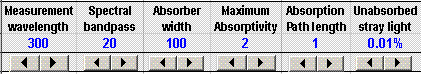The peak of the slit function falls at the wavelength setting of the monochromator. You can control the wavelength setting by using the Wavelength setting control above the graph; this is equivalent to turning the wavelength knob on the spectrometer.

The other controls above the graph are for the other variables in this simulation, such as the path length of the absorption cell (1-10 cm).  So that you can see how different types of absorbing species would behave, the simulation allows you to vary the maximum absorptivity of the analyte and the spectral width of the absorber (that is, the width of the absorption bands that constitute the absorber's spectrum).

The last control is for the stray light.  Every real monochromator passes a small amount of white light as a result of scattering off optical surfaces within the monochromator (mirrors, lenses, windows, and the diffraction grating).  Usually this so-called "stray light" is a very small fraction of the light intensity within the spectral bandpass, but it's important because it can lead to a significant source of deviation from Beer's Law.  In most cases the monochromator is tuned to the wavelength of maximum absorption of the analyte, in order to achieve the greatest sensitivity of analysis.  But that means that stray light is less absorbed than the light within the spectral bandpass.  The worst offender is stray light that is not at all absorbed by the analyte - "unabsorbed stray light", usually expressed as a percentage of the light intensity within the spectral bandpass.  In the simulation, this is set by the "Unabsorbed stray light" control.  Typical monochromators have stray light rating in the 0.01 - 1% range, depending on the wavelength setting and the type of light source used.  The stray light is always worse at wavelengths where the light source is least intense and where the detector is least sensitive. (However, in this simulation, the stray light does not automatically change with wavelength).  Note: when adjusting the stray light, use the number spinner (small arrows below the number) rather than typing directly into cell F3.  The other variables you can change either by typing or by using the number spinners.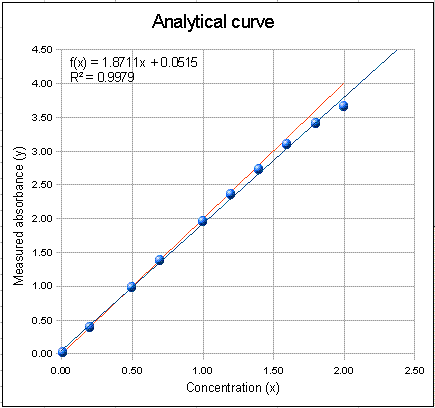The graph on the right of the window is the analytical curve (calibration curve), showing the absorbances measured for each of the standard solutions listed in the table in the top middle of the window.  You can type any set of concentrations in the concentration column of this table, up to a maximum of 10 standards. The red line in the plot (sometimes obscured by the other lines) represents the ideal Beer's Law absorbances, the blue dots represent the measured absorbances for each standard solution, and the blue line is the least-squares straight-line fit to the concentration-absorbance data.  Ideally, the fitted straight line (blue line) should go right through the middle of the blue dots.  Also on the plot is the equation of the fitted line (x = concentration and f(x) = absorbance) and the R2 value, which is a measure of the degree of correlation between absorbance and concentration (1.0000 means perfect correlation; anything less than 1.0000 is not perfect).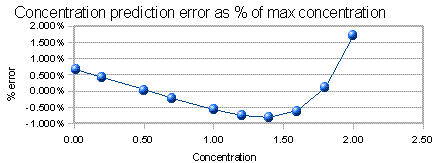The graph below the calibration curve is the concentration prediction error. If you were to run the standards as unknowns and predict their concentrations from the straight-line fit to the calibration curve, this would be the error in prediction, expressed as a percentage of the highest concentration. (The standard deviation of those errors is a good single-number summary of those errors; it is displayed to the left). This is a more sensitive indicator of non-linearity than the R2 value.

2. Start the experiment with a nearly ideal case (with the spectral bandpass much less than the absorption width and no stray light). Set wavelength = 300 nm, slit width = 10 nm, absorber width = 200 nm, maximum absorptivity = 1, path length = 1 cm, and unabsorbed stray light = 0.  Note that the ideal absorbances (red line), the measured absorbances (blue dots), and the least-squares fit (blue line) are essentially identical, even at the highest concentrations, and the R2 is exactly 1.0000, showing that the instrument readings follow Beer's law in this nearly ideal case.  You can see that in this case the absorption spectrum is almost flat over the spectral bandpass. This means that all the photons have essentially the same absorption coefficient, a fundamental requirement of Beer's Law. The concentration prediction error (the graph below the calibration curve) is so small it is negligible compared to other errors that are likely to be greater anyway, such as volumetric calibration accuracy and precision. But real-world absorption measurements are never so perfect.

3. Unabsorbed stray light limit only. Leave the absorber width = 100 nm, slit width = 10 nm, maximum absorptivity = 1, path length = 1 cm, and set the unabsorbed stray light = 0.1%, using the number spinner - small arrows below the number - rather than typing directly into cell F3. For comparison, try a stray light of 1% and 0.01% and observe the calibration curve shape. Notice that the measured absorbance bends off from a straight line at the highest concentrations, but still very linear at lower concentrations.  Why does the calibration curve flatten out at high concentrations?  Simple!  As the concentrations increases, the intensity of the transmitted light from the spectral bandpass decreases towards zero, but the unabsorbed stray light remains at the same intensity because it is unabsorbed. So eventually at very high concentrations, all that remains in the transmitted light is stray light, which results in an transmittance reading of T = (I+straylight)/(Izero + straylight), which approaches (straylight)/(Izero + straylight) as I approaches zero.  See if you can devise a rule that will predict the plateau absorbance for a given stray light percent.

4. Typical situation in solution spectrophotometry.  Set wavelength = 300 nm, slit width = 20, absorber width = 100, and leave maximum absorptivity = 1, path length = 1 cm, % stray light = 0.01% Note that analytical curve plot is almost perfectly linear (correlation coefficient is 1.0000) up to a measured absorbance of 2, yet the slope is 2% less than the ideal line (in red). In other words, just because the the analytical curve seems to be linear does not mean that the measured absorbance equals the ideal peak absorbance. (Of course, in most cases you don't really need to know the true peak absorbance, because almost all practical applications of absorption spectroscopy in chemical analysis are calibrated by using standard solutions). The concentration prediction error, based on a linear fit, is less than 0.05%. This gives an idea of the error that is caused by the slight residual non-linearly of the calibration curve.

5. Effect of changing the wavelength. Leave everything as it was, except return the the maximum absorptivity to 1.0 and the stray light to 0.01%.  Increase the wavelength setting to 350 nm and see what happens: the calibration curve plot has a lower slope, of course, because the absorptivity is less at 360 nm that at 300 nm.  But that's not all. The curve is also substantially less linear: the R2 drops to 0.9998 and the concentration prediction error goes up about 10-fold to 0.5%. Why should the calibration curve be less linear? Think about the total change in the absorptivity of the analyte over the spectral bandpass (look at how much the red line changes under the blue triangle). When the wavelength is set at a maximum (or a minimum), the total change in absorptivity over the spectral bandpass is less than when the wavelength is set to the side of a band, where the rate of change of absorptivity with wavelength is greatest. Then think about the requirement that all the photons have essentially the same absorption coefficient.  This effect is called the "polychromatic light" effect.  You can decrease the polychromatic light by decreasing the spectral bandpass (using a smaller slit width).

Note that the R2 is not a very sensitive indicator of non-linearity: even when it is just slightly less than 1.000, significant non-linearity may be present.  Looking at the concentration prediction error plot (also called the "residual" plot) is more informative that just looking at the R2 value.

You might ask why some spectrometers even have adjustable slit widths, when the best linearity and adherence to Beer's Law is observed at the smallest spectral bandpass. Why not just use the smallest slit width setting all the time? The answer is that wider slits let in more light, which improves the precision of light intensity measurement. See the simulation "Effect of Slit Width on Signal-to-Noise Ratio in Absorption Spectroscopy" at http://terpconnect.umd.edu/~toh/models/AbsSlitWidth.html for a simulation of this aspect.

6. Measuring higher concentrations at alternative wavelengths. Suppose we wanted to measure some high concentrations, above the usual linear range of the calibration curve, without diluting the samples (which would be time-consuming and possibly expensive and error prone) and without using shorter path length cells (which also involves extra cost).

To illustrate this problem, you can simply increase the maximum absorptivity from 1.0 to 2.0, which will instantly double all the absorbances.  Leave absorber width = 100, source width = 20, % stray light = 0.01 and increase the maximum absorptivity to 2.0.  With the wavelength set to the maximum at 300 nm, the linearity is not so great (R2 = 0.998; concentration prediction error = 0.78%). This is mainly because of stray light, which effects the absorbance above 3.0.

What about changing the wavelength of measurement to a less sensitive wavelength. Changing the wavelength is quick and doesn't cost anything.  But we found in #5 that measuring on the side of a band leads to a great increase in non-linearity. Set the measurement wavelength to 350 nm. This reduces the absorptivity (sensitivity) by about half. The linearity in this case is actually improved (R2 = 0.9992; concentration prediction error = 0.48%) despite the fact that the polychromatic light effect is worse at this wavelength, as you observed in #5. That's because the stray light effect is lessened by the reduced absorbance at the higher wavelength. In this case the stray light effect is greater than the polychromatic light effect.

But you can do even better than this. In this particular simulation, the absorber has a minimum in its absorption spectrum at about 225 nm. At that wavelength we have a similar sensitivity reduction, which reduces the stray light effect, but the polychromatic light effect is much less on the minimum than on the side of the sloping slide of the spectrum. Set the measurement wavelength to 225 nm. You can see that the linearity is greatly improved (R2 = 1.000; concentration prediction error = 0.023%). So the best approach is to use the peak wavelength for lower concentrations and the minimum as the alternative wavelength for higher concentrations.

7. Atomic absorption. Sometimes it is not possible or practical to have the ideal situation where the spectral bandpass is much narrower than the spectral width of the absorption.  For example, in line source atomic absorption spectroscopy, the effective spectral width of the light source is set by the line width of the hollow cathode lamp (not by the monochromator's spectral bandpass), and the absorber width is determined mainly by the temperature and pressure in the atomizer. As a result, the absorber width is only about 3 times larger than the spectral width of the light source. For example, the line width of the hollow cathode lamp might be 0.001 nm and the  absorber width might be 0.003 nm. To simulate this situation, we'll let 10 units represent 0.001 nm and set the slit width = 10, absorber width = 30 (because it's really only the ratio of the widths that is important), stray light = 0.1%, and change the absorption peak shape to Lorentzian (a better match to the shape of an atomic absorption line in an atomic absorption atomizer).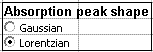With these settings, the measured absorbance is 6% less than the true value, but the linearity is fairly good (R2 = 0.9999) up to an absorbance of about 2 and the concentration prediction error is only about 0.3%.

But the situation is substantially worse if one attempts to do continuum-source atomic absorption with a medium-resolution spectrometer.  In that case the spectral bandpass might be 10 or more times larger than the absorption width. Set the absorber width = 10, slit width = 100, and leave the peak shape set to Lorentzian.  Note the linearity is substantially worse: (R2 = 0.97; concentration prediction error = 5.6%). This is one reason why continuum-source atomic absorption utilizes high-dispersion "echelle" spectrometers that can achieve a spectral bandpass about 10 to 100 times smaller than conventional monochromators at the same slit width. Note: there are other spectroscopic complexities with line-source atomic absorption: see Spectroscopic Simulation of Atomic Absorption for a more specific simulation of atomic absorption.

8. Extensions and next steps. Several extensions of this line of investigation might be taken:

a. The simulation "Effect of Slit Width on Signal-to-Noise Ratio in Absorption Spectroscopy" considers how the slit width and the dispersion on the monochromator effects the precision and signal-to-noise ratio of intensity and absorbance measurement.  b. "Signal-to-noise ratio of absorption spectrophotometry" provides a more detailed model of a UV-visible absorption spectrophotometer with a continuum source (e.g. tungsten incandescent lamp, modeled as a blackbody), dispersive monochromator, and a photomultiplier detector.

c. "Comparison of Calibration Curve Fitting Methods in Absorption Spectroscopy" compares three different methods of fitting the calibration curve non-linearity in absorption spectroscopy.

d. Two simulations consider the extension to multi-wavelength data such as would be acquired on diode-array, Fourier transform, or computer-automated scanning spectrometers:
• "The TFit Method for quantitative absorption spectroscopy", located at http://terpconnect.umd.edu/~toh/spectrum/TFit.html, describes a computational approach that eliminates the calibration curve non-linearity by basing the measurement of absorbance on a model of the actual spectroscopy of the measurement, rather than assuming that the instrument adheres to the limiting case of zero spectral bandpass and zero stray light.

Frequently Asked Questions (taken from actual search engine queries)

1. Exactly what does it mean to 'follow Beer's Law'?
Basically it means that the measured absorbance
is proportional to the concentration of the absorber, that is, a plot of absorbance vs absorber concentration is a straight line. Absorbance is defined as log(Io / I), where "Io" is the intensity of the light incident on the sample and "I" is the intensity of the light transmitted through the sample,

2. What is the equation for absorbance vs concentration?

Aalpha*L*c

where alpha is the absorption coefficient (or absorptivity), L is the path length of the light through the absorber, and c is the concentration of the absorber.  The absorbance A is defined as log(Io / I), where Io is the intensity of light beam that strikes the absorber and I is the intensity of light beam after it passes through the absorber. This is called the Beer-Lambert Law or Lambert-Beer Law or Beer-Lambert-Bouguer Law. (Strictly speaking, Beer's Law refers to the relationship of absorbance and concentration and Lambert's Law refers to the relationship of absorbance and path length, but the two are usually combined into one).

3.
Why does absorbance increase with concentration?
Because the higher the concentration, the more absorbing molecules are in the light path to absorb the light. It's like brewing tea: weak tea has a low concentration of tea dissolved in the hot water and a light color (does not absorb much light).  Strong
tea has a high concentration of tea and a darker color (absorbs lots of light).

4.
What is a Beer's Law calibration curve? How do you make and interpret a Beer's Law plot? Why are most calibration curves of Beer's Law rather than Lambert's Law?
The usual Beer's Law plot is a plot of concentration of absorber on the x (horizontal) axis, vs
measured absorbance on the y (vertical) axis. This is useful when you want to determine the concentration of solutions by measuring their absorbance. The slope of this plot is the product of the path length L times the absorption coefficient alpha where the slope is defined as the ratio of the y-axis difference to the x-axis difference between any two points on the line. (This is in contrast to a Lambert's Law plot of path length on the x axis vs measured absorbance on the y axis. This might be useful if you want to determine the path length of an absorber by measuring its absorbance. The slope of that plot would be the product of the absorptivity alpha times the absorber concentration c).

5. What are the units of the absorption coefficient, alpha?

It depends on the units of concentration and path length. If concentration is measured in moles/liter (molarity) and path length in cm, then the units of the absorption coefficient (also called the molar absorptivity) are liters/mole-cm. If concentration is measured in grams per liter and path length in cm, then the units of the absorption coefficient are liters/gram-cm. If concentration is measured in grams per mL (cubic centimeters) and path length in cm, then the units of the absorption coefficient are mL/gram-cm.

6. How do you know
the value of the absorption coefficient, alpha?
Absorption coefficients are determined experimentally and are tabulated for a large number of compounds in chemistry reference works. If you solve the above equation for alpha, you get:

alpha = A / (L*c)

So by measuring the absorbance A of a known concentration c of the absorbing compound using an absorption path length L, you can calculate alpha. Because A has no units (it's the log of a ratio of two intensities, so the intensity units cancel out), and because L and c are in the denominator, the units of alpha are the reciprocal of the units of  L and c.

Absorption coefficients vary widely from substance to substance and also vary with wavelength. Values of alpha are tabulated in the literature and in reference books for many common molecules.

7. What's the difference between 'absorption' and 'transmission'?
Absorption refers to how much light is lost when passing through an absorber. Transmission refers to how much light remains after it passes through.
Absorption is expressed as the absorbance, log(Io / I) or as the absorption(Io-I) / Io, or the percent absorption, 100(Io-I) / Io. Transmission is expressed as the transmittanceI / Io, or as the percent transmission, 100(I / Io). As the absorber concentration goes up, the absorbance and the absorption both go up, but the transmission goes down. Of these, absorbance is the most widely used because it is directly proportional to concentration, according to Beer's Law. Note that all of these measures are based on the RATIO of the two intensities Io and I. This has the huge advantage of making these measures independent of the overall intensity of the light source and of the sensitivity of the detector used to measure the intensity. This in turn helps to make these quantities independent of the instrument used to measure it.

8. Why does the Beer-Lambert Law lead to absorbances above 1?
An absorbance of 1 simply means that the transmitted intensity, I, is one-tenth of the incident intensity,
Io. If the transmitted intensity is lower than that, the absorbance is higher than 1. You might be confusing absorbance with absorption. As the absorber concentration goes up, the absorbance, log(Io / I), and the absorption, (Io-I) / Io, both go up (and the transmission goes down), but the absorption can't get any higher than 1, whereas the absorbance keeps going up proportional to concentration. You can easily get absorbances above 1, even up to 3 or 4 under ideal conditions.

9. How do you measure unknown concentrations with absorption spectrophotometry? Is it better to use a standard curve or the equation for Beer's Law?
If you solve Beer's Law for concentration, you get:
c = A / (L*alpha)

So, you could determine the concentration c simply by measuring the absorbance A and dividing it by the product of the path length L and absorptivity alpha. That is, if you know alpha. Values of alpha are tabulated for many common molecules, but the trouble is that alpha varies as a function of wavelength, temperature, solvent, pH, and other chemical conditions, so if the conditions of your sample don't match those with which the alpha was measured, the calculated concentration won't be accurate. Also, some spectrophotometers do not follow Beer's Law exactly; it's not uncommon for some instruments to give absorbance readings that are a little too low and slightly non-linear with respect to concentration. Because of this, it's better to prepare a series of standard solutions of known concentration, made up so that the chemical conditions are as close as possible to those of the sample, measure their absorbances on your instrument, and plot a calibration curve with concentration of the standards on the x-axis vs measured absorbance on the y-axis. Once the calibration curve is established, unknown solutions can be measured and their absorbances converted into concentration using the calibration curve. Here is a graphic animation of this calibration process applied to a specific assay. This can be done either graphically (by drawing a line from the absorbance of each unknown across to the calibration curve and then down to the concentration axis) or it can be done mathematically (by fitting a line or curve to the calibration data, solving the equation of that line for concentration, then using that equation to convert measured absorbances to concentration). With computers, it's usually easier to do the latter. The important point is that even if Beer's Law is not obeyed perfectly, you can still get accurate resulting using a calibration curve.

10
.
How do you solve Beer's Law for transmittance? Does graphing transmission or absorbance result in a more accurate standard curve? Why is a straight line calibration better?
If you state Beer's Law as
I = Io*10-(alpha*L*c), then just divide both sides of the equation by Io, the result is I / Io = 10-(alpha*L*c) = 10-A, where A is absorbance. The quantity I / Io is defined as transmittance. Absorbance A is defined as log(I / Io). In principle, either transmittance or absorbance would work equally well for quantitative analysis, because there is exactly the same amount of information in an transmission reading as in an absorbance reading; one can be converted to the other without loss. However, a calibration curve plotted in absorbance is linear, according to Beer's Law, whereas a calibration curve plotted in transmission would be highly non-linear (exponential, in fact). It's just easier to fit a line to a set of straight-line data, and to see when the data are deviating from that straight line, that to a fit a curved line to non-linear data, whether it is done by hand or with a calculator or computer.

11. What are the limitations of beer's law?  What limits the linearity of Beer's Law plot?
Deviations from Beer's Law can be caused by:
(a) Stray light, which is any light striking the detector whose wavelength is outside the spectral bandpass of the monochromator or which has not passed through the sample;
(b) Polychromatic light effect, which occurs if the absorber's absorption coefficient alpha varies over the wavelength interval of light passing through the sample;
(c) unequal light path lengths across the light beam;
(d) unequal absorber concentration across the light beam;
(e) changes in refractive index of the solution at high analyte concentration;
(f) light-scattering by the sample matrix, especially in turbid samples, resulting in a significant  absorption signal even when the absorber's concentration is zero;

(g) shifts in chemical equilibrium involving the absorber as a function of concentration;
(h) changes in pH as a function of concentration.

(i) fluorescence of the absorber, in which some of the absorbed light is re-emitted and strikes the detector;
(j) chemical reactions caused by the absorption of light, including photolysis, dimerization, polymerization, and molecular phototropism (change in molecular shape when the molecule absorbs light).
(k) if the light intensity is extremely high (like a focused laser), it's possible to observe non-linear optical effects, which are a fundamental failure of the Beer-Lambert Law.

The most common of these are (a) and (b), which both result in a concave-down curvature of the Beer's Law plot; (c) and (d) are easily avoided by proper experiment and instrument design (square cuvettes, well-mixed solutions); (e) is only a problem at very high concentrations; (f) is pretty common in real-world applications to complicated samples, but can be minimized by special measurement techniques and instrument designs
; (g) and (h) can be avoided by buffering the solutions to constant pH and adjusting the concentration of reagents; (i) and (j) occur rarely with some particular absorber molecules and must be treated on a case-by-case basis; (k) never occurs in standard laboratory instruments with conventional light sources..

12. Under what conditions is the Beer-Lambert law not obeyed?
The Beer-Lambert Law will not be obeyed if the photons of light striking the detector do not all have an equal chance of absorption by the sample. This can happen if they have different absorption coefficients, different path lengths through the sample, or if they encounter different concentrations of sample molecules. Also if anything else is present in the sample that absorbs light or causes light scattering, the measured absorbance will not be zero when the analyte's concentration is zero, contrary to Beer's Law. If the absorber undergoes any type of chemical reaction or equilibrium that varies as a function of concentration, Beer's Law will not be obeyed with respect to the overall or total concentration, because the concentration of the actual absorbing molecule is not proportional to the overall concentration of the solution. The "c" in Beer's Law refers to the concentration of just the absorber, not to the total concentration of all the compounds reacting with or in equilibrium with the absorber. Even if Beer's Law holds exactly for each individual compound, the total absorbance of the mixture will not follow Beer's Law with respect to the total concentration if the proportion of each compound changes with concentration (unless by chance the absorptivity of all those compounds happens to be exactly the same).

13. Why are measurements taken in increasing order of concentration when using spectrophotometry?
Mostly it's just a convention. Actually, the measurements can be taken in any order; if the instrument and the samples and standards are stable with time, the result will be essentially the same.
The one situation where the order of measurement is done from lowest to highest concentration is if the sample cuvette is difficult to clean thoroughly once it is exposed to high concentrations.

14. What happens to the energy of the absorbed light be
measured?  What about the "Law of Conservation of Energy"?
Conservation of energy still works. The energy of the absorbed light is converted into heat, which increases the temperature of the measured samples slightly. But in an ordinary instrument the temperature increase is very small and not even easily measurable.

15. How can the intensity of absorbed light be measured?
In absorption spectrophotometry the absorbed light intensity is not measured directly, rather it's measured indirectly by measuring the difference between the incident and transmitted intensity.

16. I see how the detector measures the transmitted intensity, I. How does it measure the incident intensity, Io?
If you remove the sample from the light beam, the detector then measures the incident intensity,
Io, because there is noting in the beam to absorb light (except air). But for the measurement of solution samples contained in sample cells (cuvettes), there is an additional complication: the cuvette itself reduces the light intensity, by light reflection from the surfaces of the glass, with the result that an empty cuvette would give a significant absorption signal. Also, sometimes the solvent absorbs some of the light. To compensate for both of these effects, you need to measure a cuvette filled with solvent (which is called the "blank"), and subtract the absorbance of the blank from all the standards and samples. This effectively subtracts out the absorption of the cuvette and solvent, and the resulting difference is the absorbance of the analyte alone.

17. How do you use spectrophotometry to measure things that are colorless?
It is very common in analytical spectrophotometry to use a "color reagent" that will react with a colorless analyte under appropriate conditions to produce a stable colored product that absorbs in the visible, preferably at a wavelength where other components in the sample do not absorb. There are a large number of such reagents commercially available to meet many analytical requirements. Another possibility is that many colorless compounds absorb in the ultra-violet (uv) region from 200 nm - 400 nm. If the other components in the samples do not absorb significantly in the uv, a spectrophotometric analysis in the uv region possible. But to do this you must use a uv-visible spectrometer, and cuvettes (usually quartz or fused silica) which are transparent in the uv. As a solvent, water is quite transparent in the uv, but if you must use another solvent, make sure its uv absorption at the analytical wavelength is not too much.

18. Why does the Beer-Lambert Law require monochromatic light?
Actually the Beer-Lambert Law requires that all
the photons of light striking the detector have an equal chance of absorption by the analyte. This requires that all the photons have the same absorption coefficient, which will be the case either if they all have the same wavelength (i.e. monochromatic light) or if the sample absorption is constant over the wavelength range of the light beam (e.g. at the maximum or minimum of a broad absorption peak).

19. What is the low concentration limit of Beer's Law?
There is no fundamental low concentration limit, but at very low concentrations, the readings of absorbance can be in error due to the limited resolution of the readout display or because of the signal-to-noise ratio of the light intensity measurement (due to detector nose, photon noise, or light source fluctuation).

20. What is the high concentration limit of Beer's Law? What is the approximate concentration above which deviation from Beer's Law first become apparent?
Normally, above whatever concentration produces an absorbance of about 2, deviations start to become apparent. Stray light especially becomes more important at
high absorbances. Low-quality instruments, especially when operated near their wavelength limits, exacerbate the non-linearity at high absorbances.

21. What is the instrument measurement range of transmission in absorption spectrophotometry?  What is the optimum transmittance range for optimum precision?  The measurement range of good-quality instruments is typically from an
absorbance of about 0.0001 (transmittance = 0.9998 or 99.98 %T) to an absorbance of about 4 (transmittance = 0.0001 or 0.01%). The best precision of concentration measurement occurs at about an absorbance of 0.5 to 1.0 (10 to 30%T), depending on what exactly is the dominant source of random noise. This is demonstrated in the simulation Effect of Slit Width on Signal-to-Noise Ratio in Absorption Spectroscopy.

22. Why are absorbance readings taken at the peak wavelength of maximum absorbance?  Must you always use the maximum? What is the wavelength of least error?
It really depends on what is the largest source of error. Taking the readings at the peak maximum is best at low absorbances because it gives the best signal-to-noise ratio, which improves the precision of measurement.
If the dominant source of noise is photon noise, the precision of absorbance measurement is theoretically best when the absorbance is near 1.0. So if the peak absorbance is below 1.0, then using the peak wavelength is best, but if the peak absorbance is well above 1.0, you might be better off using another wavelength where the absorbance is closer to 1. Another issue is calibration curve non-linearity, which can result in curve-fitting errors. The non-linearity caused by polychromatic light is minimized if you take readings at either a peak maximum or a minimum, because the absorbance change with wavelength is the smallest at those wavelengths. On the other hand, using the maximum increases the calibration curve non-linearity caused by stray light. Very high absorbances cause two problems: the precision of measurement is poor because the transmitted intensity is so low, and the calibration curve linearity is poor due to stray light. The effect of stray light can be reduced by taking the readings at a wavelength where the absorbance is lower or by using a non-linear calibration curve fitting technique. Finally, if spectral interferences are a problem, the best measurement wavelength may be the one that minimizes the relative contribution of spectral interferences (which may or may not be the peak maximum). In any case, don't forget: whatever wavelength you use, you have to use the exact same wavelength for all the standards and samples.

23. How can you identify the cause of the deviation from Beer's Law
There are two experiments you can perform that will throw at least some light on this question (pardon the pun). First, measure the absorbance of a single concentration at different path lengths (by using different sample cuvettes) and plot the measured absorbance vs path length (this is a Lambert's Law plot). Second, prepare a series of standard solutions of different concentrations, measure then in a fixed path length, and plot concentration vs absorbance
(this is a normal Beer's Law plot). Fit a straight line to both of these sets of data. If the Lambert's Law plot is non-linear (concave down), then the problem is optical rather than chemical, most likely polychromatic light or stray light. If the plot is linear at low concentrations but non-linear at high concentrations, it's probably stray light. If the Lambert's Law plot is linear, but the Beer's Law plot is non-linear, it suggests that the non-linearity is chemical in nature, perhaps an equilibrium shift that depends on the concentration of the solution.

24. How can you distinguish between random and systematic deviation from Beer's Law?  How can I locate the non-linear region?
Fit a straight line to the calibration data and look at a plot of the "residuals", the differences between the y values in the original data and the y values computed by the fit equation
. Deviations from linearity will be much more evident in the residuals plot than in the calibration curve plot. (Click here for a fill-in-the-blank OpenOffice spreadsheet that does this for you. View screen shot). If the residuals are randomly scattered, then it means that the deviations are caused by random errors such as photon or detector noise or random volumetric or procedural errors. If the residuals have a smooth shape, this means that the errors are systematic. If the residual plot has a straight line segment at low concentrations but curves off at high concentrations, then it's probably stray light that is causing the non-linear region.

25. What is the minimum
value of the coefficient of determination (R2to obey Beer's Law?
It depends on the accuracy required. As a rough rule of thumb, if you need an accuracy of about 0.5%, you need an R2 of 0.9998; if a 1% error is good enough, an
R2 of 0.997 will do; and if a 5% error is acceptable, an R2 of 0.97 will do. The bottom line is that the R2 must be pretty darned close to 1.0 for quantitative results in analytical chemistry. But if the deviation from linearity is smooth and gradual, rather than random, you can still get accurate results with a "curvilinear" calibration curve fitting technique, such as a quadratic or cubic fit.

26. What does it mean if the calibration curve (Beer's Law plot) does not extend through zero?
If it's close to zero, it may simply be due to random error in the absorbance readings or the volumetric preparations. Another possibility is that the calibration curve shape is curved, but you have fitted a straight line to it; use a non-linear curve fit instead).  Finally, it may mean that you have not properly subtracted the "blank" (see #16, above).

27. What is the effect of the slit width on the spectra in uv-vis spectrophotometry?
The slit width determines the spectral bandpass, the wavelength range of the light passing through the sample. The smaller the slit width, the more nearly monochromatic the light beam will be. But if the slit width is too large, the polychromatic light effect will cause the spectral peaks to be shorter and broader than they would be at narrower slit widths. If you are trying to measure an accurate absorption spectrum, for example for use as a reference spectrum for future measurements or for identification of that absorber, then you should use a narrow slit. However, the signal-to-noise ratio decreases as the slit width is reduced, so it is not always practical to use the smallest slit width possible. If the spectral bandpass is one tenth (1/10th) of the spectral width (full width at half-maximum) of the narrowest band in the spectrum, then the maximum peak height error caused by polychromaticity will be less than 1%.

28. How does Beer's Law apply to atomic absorption spectroscopy?
Beer's Law is the basis of atomic absorption spectroscopy, as it is for conventional molecular spectrophotometry. But there is a big difference. In atomic absorption, the absorbers are free atoms in the gas phase in a high-temperature flame or graphite furnace atomizer, and their absorption spectra consist of very narrow spectral "lines", only about 0.003 nm in width (compared to a typical molecule in solution that might have a spectral width of 50 - 100 nm or more). So in order for Beer's Law to be obeyed with such an extremely narrow absorption, you would to use a light beam with an even narrower spectral width, ideally much less than 0.003 nm. Ordinary monochromators can not achieve a spectral bandpass anywhere near that narrow, so a conventional optical design is impossible. The problem is solved in two distinctly different ways. The most common and least expensive type of
atomic absorption instrument uses an atomic vapor lamp as the primary light source, which emits the atomic line spectrum of the element to be determined. A small monochromator is used, positioned between the flame or graphite furnace and the detector, but its function is only to isolate one line from the line source and to reduce stray light. The line width of the light source is typically about 0.001 nm or so, not very much less than the absorption line width, so adherence to Beer's Law is not perfect, but it's good enough at low concentrations. The disadvantage is that you need to purchase a separate lamp for each element you intend to measure. The other type of instrument uses a continuum source, a special type of high-resolution spectrometer called an "echelle" spectrometer, and a diode-array detector. The advantage of this approach is the ease of switching from one element to the next and the possibility of simultaneous multi-element measurement. However, a continuum source instrument is much more expensive.

29. Why is it important not to have fingerprints on the cuvette?
Fingerprints absorb and scatter light slightly, even though they might not be readily visible. So a cuvette with fingerprints on it will give a slightly higher absorbance reading that a clean one.  Unless you compensate for this by by using the same cuvette, with the same exact fingerprint, for the blank solution, and subtract the blank signal from the samples, the measured concentration will be inaccurate.  This is especially important if the absorbance is low (say, below 0.01 absorbance).

30. Why is absorbance not measured directly?
In absorption spectroscopy,
the intensity of the absorbed light can not measured directly because the absorbed light is converted into heat, but the resulting temperature increase is far too small to be readily measured without very specialized and expensive equipment. The only thing that can be measured directly is the intensity of the transmitted beam. Making a calibration curve based on the intensity of the transmitted beam result not a good idea because the relationship to concentration is highly non-linear.

(c) 1991, 2015. This page is part of Interactive Computer Models for Analytical Chemistry Instruction, created and maintained by Prof. Tom O'Haver , Professor Emeritus, The University of Maryland at College Park.  Last updated August 2015. Comments, suggestions and questions should be directed to Prof. O'Haver at toh@umd.edu.
Number of unique visits since May 17, 2008: